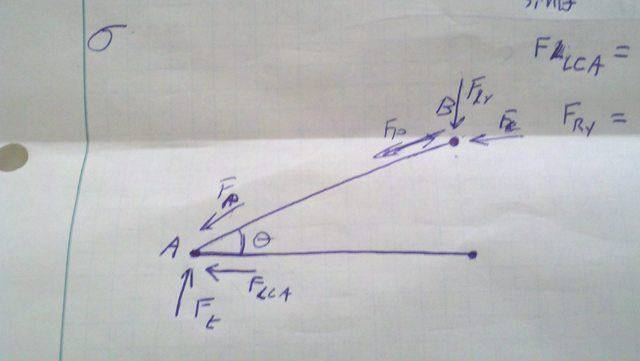# Racecar Statics and Mechanics of Solids problem

## Homework Statement

So I am designing the suspension for a small formula car. It's a pushrod suspension much like what is shown below:
[PLAIN]http://f1-dictionary.110mb.com/Images/pullrod_poshrod_push.gif [Broken]
I am trying to find the required tubing diameter for the loads the car will experience.

Ʃf(x)=0
Ʃf(y)=0

σ=F/A

## The Attempt at a Solution

The free body diagramThe car is assumed to weigh 700lbs, have 60-40 front to rear weight distribution and be in a 2G turn, so the maximum force of the tire is 525lb. θ is assued to be 45°

By the method of joints:

At pinned joint A
Ʃf(x)=0=FLCA-cos(θ)Fp
Ʃf(y)=0=Ft-Fpsin(θ)

At pinned joint B
Ʃf(x)=0=-Fs-Fpcos(θ)
Ʃf(y)=0=-FRy-Fpsin(θ)

So Fp=Ft/sin(θ)

And the maximum force in the pushrod is around 743lb. One of the tubing thicknesses under consideration is 5/8 .035 4130 chromoly which has a yield strength of 70PSI.

Cross sectional area is
∏/4(.625in-.59in)=.0275 in2

Calculating the stress at maximum load is calculated at around 27000 PSII know my statics is rusty but this is wayyyyy off. This tubing thickness is within the range of what other people are using, maybe a bit on the small side. Can anyone point out where I may have gone wrong?

Last edited by a moderator:

SteamKing
Staff Emeritus